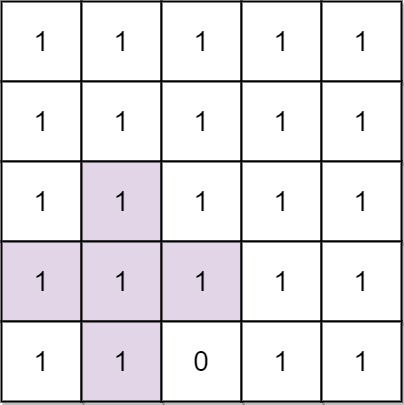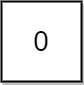# GeetCode Hub

You are given an integer `n`. You have an `n x n` binary grid `grid` with all values initially `1`'s except for some indices given in the array `mines`. The `ith` element of the array `mines` is defined as `mines[i] = [xi, yi]` where `grid[xi][yi] == 0`.

Return the order of the largest axis-aligned plus sign of 1's contained in `grid`. If there is none, return `0`.

An axis-aligned plus sign of `1`'s of order `k` has some center `grid[r][c] == 1` along with four arms of length `k - 1` going up, down, left, and right, and made of `1`'s. Note that there could be `0`'s or `1`'s beyond the arms of the plus sign, only the relevant area of the plus sign is checked for `1`'s.

Example 1:```Input: n = 5, mines = [[4,2]]
Output: 2
Explanation: In the above grid, the largest plus sign can only be of order 2. One of them is shown.
```

Example 2:```Input: n = 1, mines = [[0,0]]
Output: 0
Explanation: There is no plus sign, so return 0.
```

Constraints:

• `1 <= n <= 500`
• `1 <= mines.length <= 5000`
• `0 <= xi, yi < n`
• All the pairs `(xi, yi)` are unique.

class Solution { public int orderOfLargestPlusSign(int n, int[][] mines) { } }